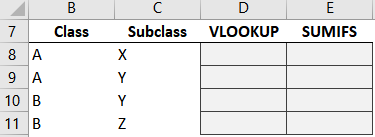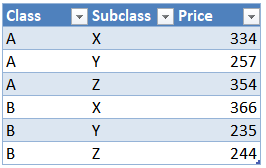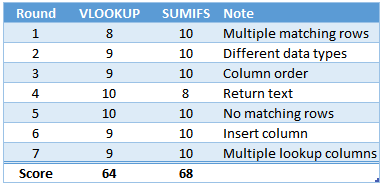# Battle of Heavyweights: Round 7The purpose of this round is to examine the issue of multiple lookup columns. Often, we have a single unique ID that we can use as the lookup value. When that is true, great. But, what happens when we need to use multiple columns for our lookup value? That is exactly what this round is all about. So let’s get to it.

## Round 7

To perform our lookup, we need to consider both the Class and Subclass values:We are trying to retrieve Price from our table (Table1):First, with VLOOKUP.

## VLOOKUP

We write the following formula into D8:

`=VLOOKUP(B8, Table1, 3, 0)`

We fill the formula down, and hmmm…A bunch of repeated values. Why? Because it is using the Class column only. That is, VLOOKUP supports a single lookup column and it is expecting it to be in the first (left-most) column in the lookup range.

Now, if we really wanted to use VLOOKUP here, we could combine both the lookup value and lookup columns into a single unique value using a variety of techniques, including CONCAT, CONCATENATE, &, TEXTJOIN, FlashFill, PowerQuery, and more.

But, let’s see if SUMIFS needs any assistance …

## SUMIFS

We write the following formula into E8:

`=SUMIFS(Table1[Price], Table1[Class], B8, Table1[Subclass], C8)`

We fill it down:Yes … it worked!

The formula is basically saying add up the values in the price column, but, only include those rows where the class column is equal to our call, AND WHERE the Subclass column is equal to our Subclass.

In other words, SUMIFS is built specifically to support multiple conditions, that is, multiple lookup columns.

Time to analyze the round.

## Analysis

In this round, we wanted to explore the idea of multiple lookup columns. So, which one would we use in practice? Well, using SUMIFS would take less effort because it is able to work with the data as it comes. In other words, it doesn’t require an extra step such as creating helper columns or combining values. It is designed specifically to support multiple conditions. So, we gotta give this round to SUMIFS.

But, how about VLOOKUP … can it do this? Yes, with the assistance of some friends such as CONCAT, TEXTJOIN, CONCATENATE, &, Power Query, and so on. So, we award 9 points to VLOOKUP.